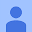# Fraction Calculator + Math

4.5
15.6K reviews
1M+Everyone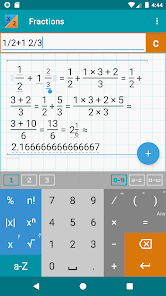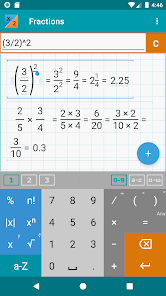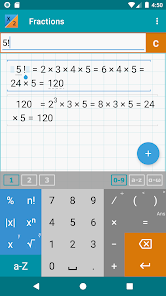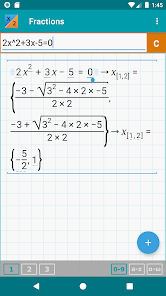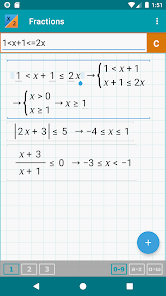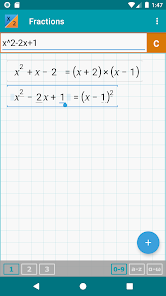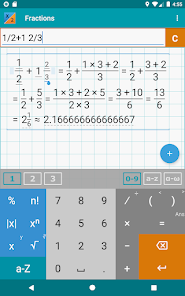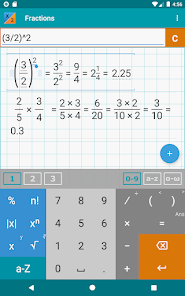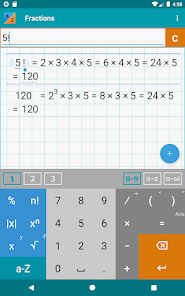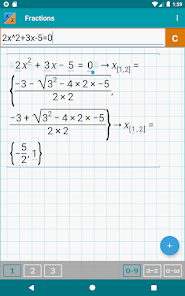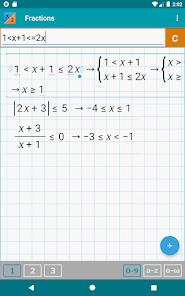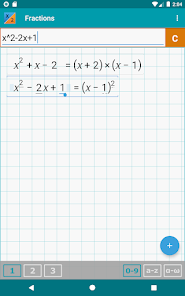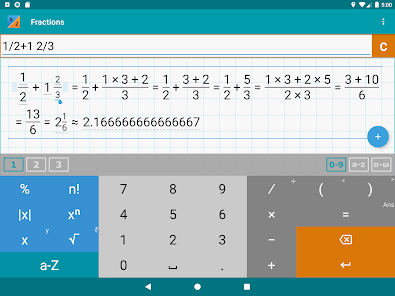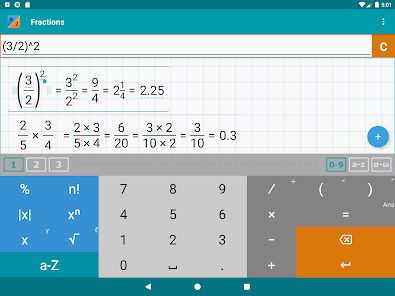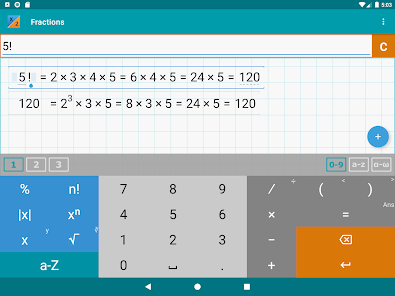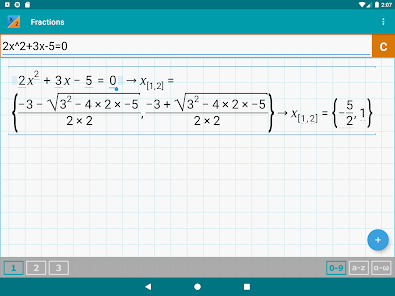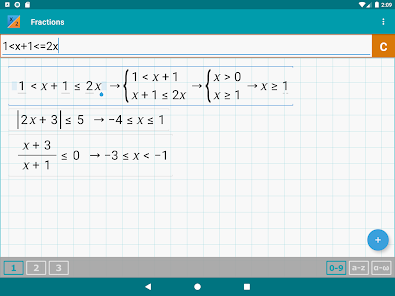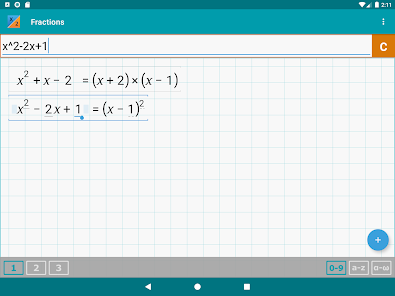Fraction calculator with step-by-step operations and algebra. Shows results as you type. Use space to enter mixed fractions. Graphical display of expressions with history.

Help site with instructions and examples: http://frac.mathlab.us
If you have a question, send email to calc@mathlab.us

FRACTION CALCULATOR
* Step-by-step operations (option to turn off)
* Arithmetic operations (+,-,*,/,÷), hold / to enter ÷
* Powers of fractions
* Fractions simplification
* Fractions with complex numbers
* Decimal to fraction conversion and back
* Symbolical fractions and operations
* Integer roots simplification
* Pinch to zoom

ALGEBRA
* Linear equations x+1=2 -> x=1
* Quadratic equations x^2-1=0 -> x=-1,1
* Approximate roots of higher polynomials
* Systems of linear equations, write one equation per line, x1+x2=1, x1-x2=2
* Polynomial long division
* Polynomial expansion, factoring
* Solving inequalities with one variable, double tap ( and ) to enter less and greater signs
* Linear and polynomial inequalities, x^3-4>4
* Inequalities with absolute values, abs(2x+3)<=5
* Compound inequalities, 1* Rational inequalities, (x+3)/(x-1)<=0
Updated on
May 27, 2023

## Data safety

Safety starts with understanding how developers collect and share your data. Data privacy and security practices may vary based on your use, region, and age. The developer provided this information and may update it over time.This app may share these data types with third parties
Device or other IDsThis app may collect these data types
App activity, App info and performance, and Device or other IDs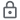Data is encrypted in transit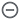Data can’t be deleted

## Ratings and reviews

4.6
14.3K reviews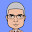Anthony C
January 7, 2023
It's different, and since I'm not familiar with that calculator style of calculating. It would be hard for me to use it. It could use a fixed decimal place option. Left and right arrows have replaced the enter button. So there is no way to calculate the answer manually when "Show Answer" is Off in settings.
1 person found this review helpful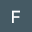Fearless KeepersOfFaith
June 29, 2023
Perfect calculator. Easy to use and very practical.
1 person found this review helpful